## The Dirac Equation

To proceed toward a field theory for electrons and quantization of the Dirac field we wish to find a scalar Lagrangian that yields the Dirac equation. From the study of Lorentz covariants we know thatis a scalar and that we can form a scalar from the dot product of two 4-vectors as in the Lagrangian below. The Lagrangian cannot depend explicitly on the coordinates.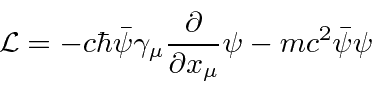(We could also add a tensor term but it is not needed to get the Dirac equation.) The independent fields are considered to be the 4 components ofand the four components of. The Euler-Lagrange equation using theindependent fields is simple since there is no derivative ofin the Lagrangian.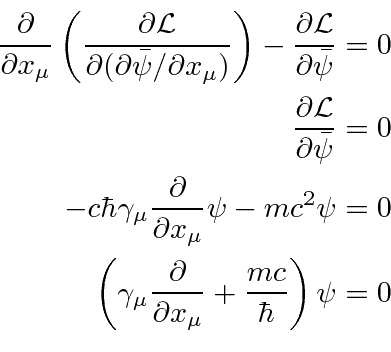This gives us the Dirac equation indicating that this Lagrangian is the right one. The Euler-Lagrange equation derived using the fieldsis the Dirac adjoint equation,

The Hamiltonian density may be derived from the Lagrangian in the standard way and the total Hamiltonian computed by integrating over space. Note that the Hamiltonian density is the same as the Hamiltonian derived from the Dirac equation directly.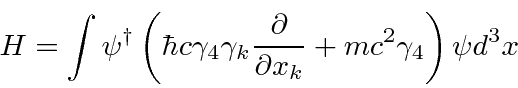We may expandin plane waves to understand the Hamiltonian as a sum of oscillators.Writing the Hamiltonian in terms of these fields, the formula can be simplified yielding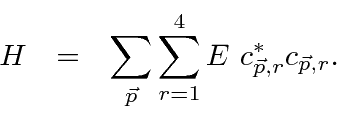By analogy with electromagnetism, we can replace the Fourier coefficients for the Dirac plane waves by operators.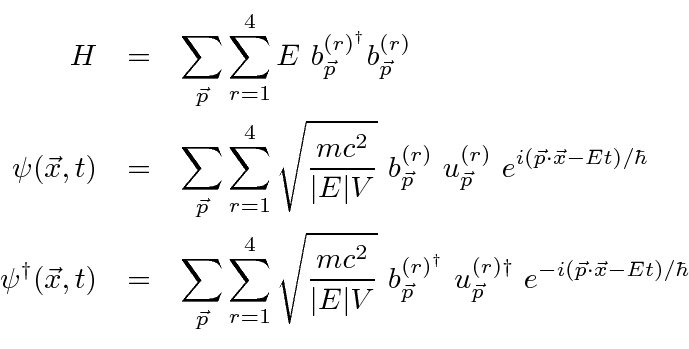The creation an annihilation operatorsandsatisfy anticommutation relations.is the occupation number operator. The anti-commutation relations constrain the occupation number to be 1 or 0.

The Dirac field and Hamiltonian can now be rewritten in terms of electron and positron fields for which the energy is always positive by replacing the operator to annihilate a negative energy state'' with an operator to create a positron state with the right momentum and spin.These anti-commute with everything else with the exception thatNow rewrite the fields and Hamiltonian.All the energies of these states are positive.

There is an (infinite) constant energy, similar but of opposite sign to the one for the quantized EM field, which we must add to make the vacuum state have zero energy. Note that, had we used commuting operators (Bose-Einstein) instead of anti-commuting, there would have been no lowest energy ground state so this Energy subtraction would not have been possible. Fermi-Dirac statistics are required for particles satisfying the Dirac equation.

Since the operators creating fermion states anti-commute, fermion states must be antisymmetric under interchange. Assumeandare the creation and annihilation operators for fermions and that they anti-commute.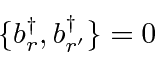The states are then antisymmetric under interchange of pairs of fermions.Its not hard to show that the occupation number for fermion states is either zero or one.

Note that the spinors satisfy the following slightly different equations.Jim Branson 2013-04-22